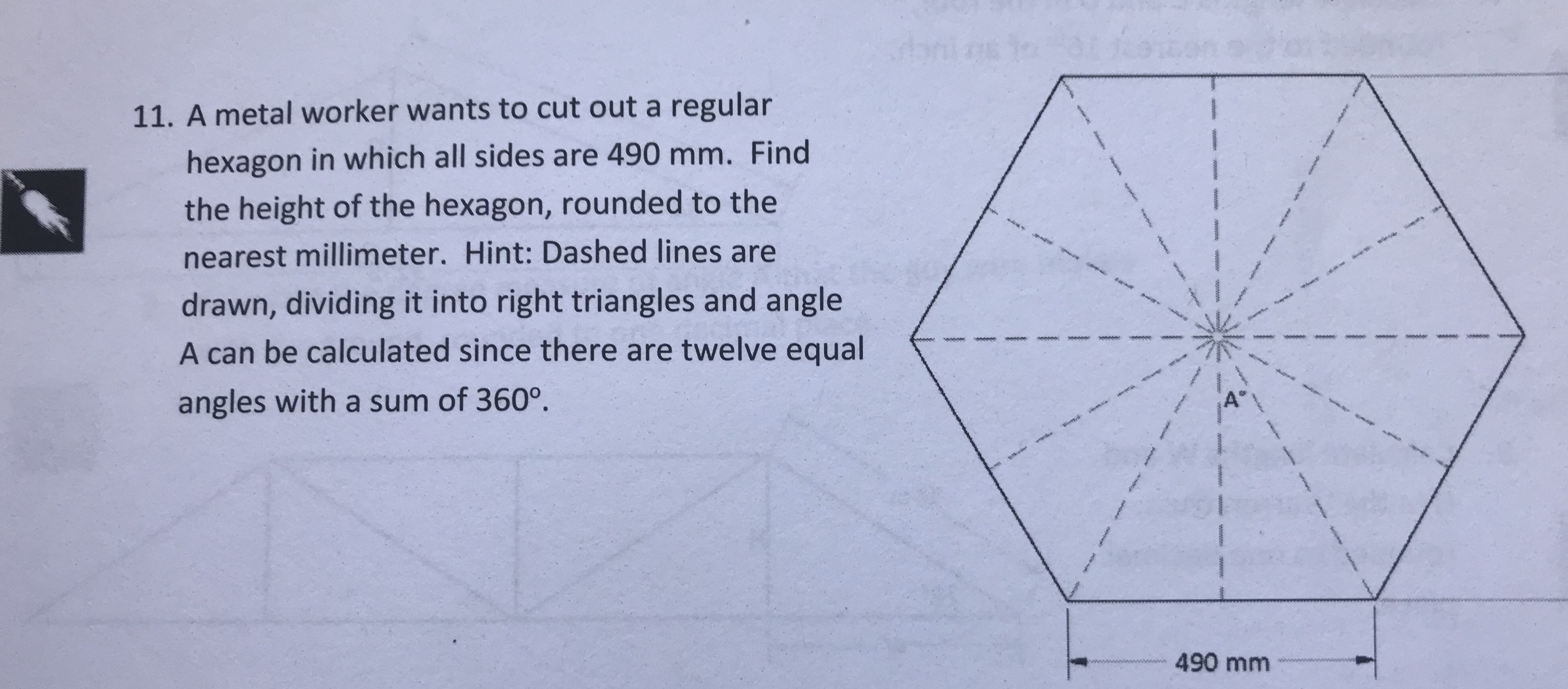11. A metal worker wants to cut out a regularhexagon in which all sides are 490 mm. Findthe height of the hexagon, rounded to thenearest millimeter. Hint: Dashed lines aredrawn, dividing it into right triangles and angleA can be calculated since there are twelve equalangles with a sum of 360°490 mm

Question

Please explain the solution in a clear manner, step by step.help_outlineImage Transcriptionclose11. A metal worker wants to cut out a regular hexagon in which all sides are 490 mm. Find the height of the hexagon, rounded to the nearest millimeter. Hint: Dashed lines are drawn, dividing it into right triangles and angle A can be calculated since there are twelve equal angles with a sum of 360° 490 mm fullscreen
Step 1

We have to find height of regular hexagon with given information.

Information is given below:

Step 2

As we know that dashed lines are dividing into right triangles and angles it makes an 12 angles with sum of all 12 angles is 360.

with the help of this we will find value of angle A.

Work is shown below:

Step 3

Now we have triangle ADC and ADB  are right angle triangle with angle D is 90 degree .

W...

Want to see the full answer?

See Solution

Want to see this answer and more?

Our solutions are written by experts, many with advanced degrees, and available 24/7

See Solution
Tagged in

Trigonometry